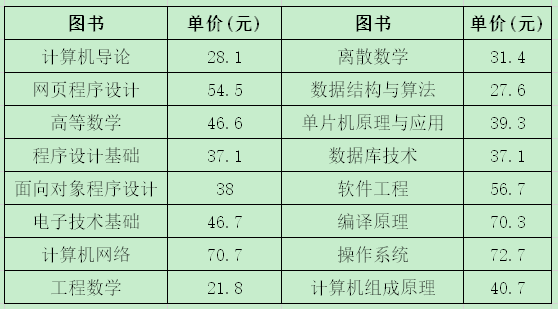2020-05-04 23:49

# 请问这个C语言的这个代码怎么做1 5 8 10 5 1 1 2 3 4 6 12 7 13 20 11

72.7 20

``````#include <stdio.h>
int main()
{
int i=0,j,m,t,a;
float p=0,b={28.1,54.5,46.6,37.1,38,46.7,70.7,21.8,31.4,27.6,39.3,37.1,56.7,70.3,72.7,40.7},c;
while(i<16){
scanf("%d",&a[i]);
i++;
}
for(i=0;i<16;i++)
{
c[i]=a[i]*b[i];
}
i=0;
for(j=1;j<=16;j++){
if(c[i]>c[j])
i=i;
else
i=j;
}
printf("%.1f %d",b[i],a[i]);
return 0;
}

``````

• 点赞
• 写回答
• 关注问题
• 收藏
• 邀请回答